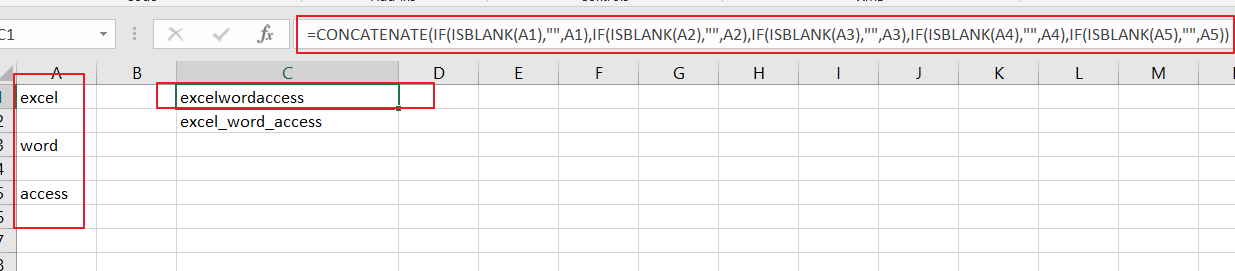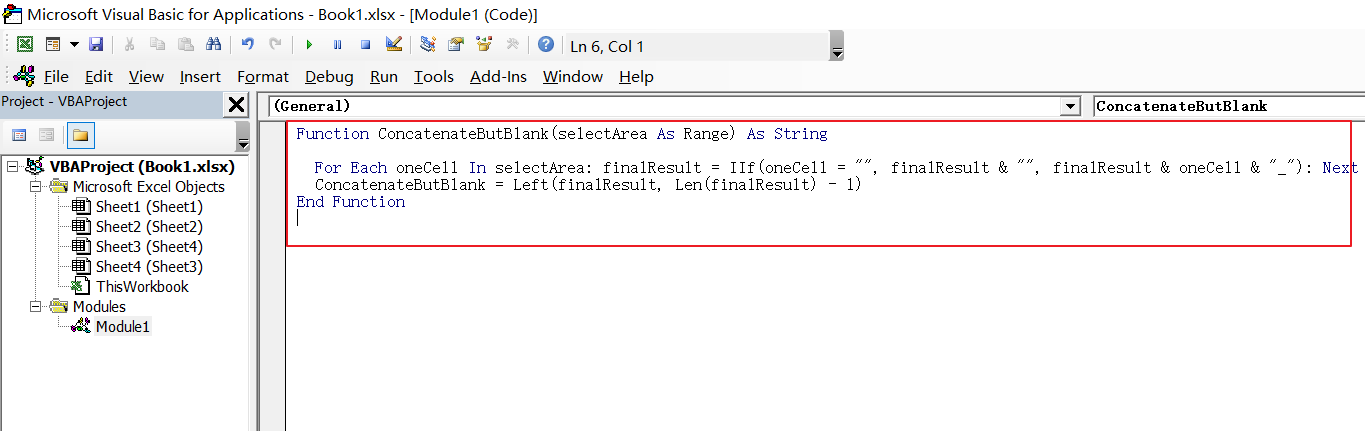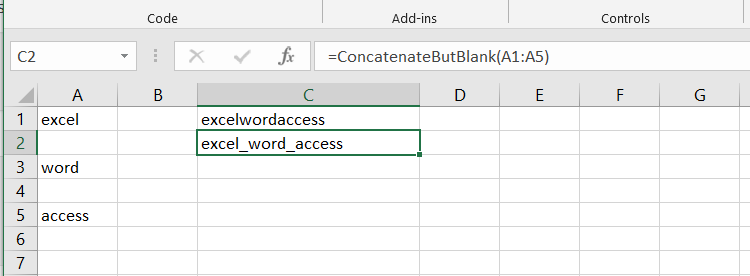# How to ignore Blank Cells while Concatenating cells in Excel

This post will guide you how to concatenate cells but ignore all blank cells in your worksheet in Excel. How do I concatenate cells but ignore blank cells with a formula in Excel. How to create a concatenate formula to skip blank cells with user defined function in Excel 2013/2016.

Assuming that you have a list of data in range A1:A5 with one or two blank cells, and you want to concatenate those cells with a formula based on Concatenate function but ignore all blank cells. By default, the Concatenate function will combine all selected cells as well as those two blank cells. The below two methods will show you how to concatenate cells but skip all blanks.

## Concatenating Cells but Ignore Blanks with a formula

To concatenate cells but ignore all blank cells in Excel, and you need to use a condition function to check whether the cell has a value or is empty cell. And if the cell is blank, then return nothing; otherwise, return the value of the cell. So you can use the below formula based on the concatenate and the ISBLANK function.

`=CONCATENATE(IF(ISBLANK(A1),"",A1),IF(ISBLANK(A2),"",A2),IF(ISBLANK(A3),"",A3),IF(ISBLANK(A4),"",A4),IF(ISBLANK(A5),"",A5))`

Type the above formula into a blank cell where you want to place the last result, and then press Enter key.## Concatenating Cells but Ignore Blanks with User Defined Function

There is another simple way to concatenate cells but skip all blank cells. And you can create one user defined function with VBA Macro code. Just do the following steps:

#1 open your excel workbook and then click on “Visual Basic” command under DEVELOPER Tab, or just press “ALT+F11” shortcut.#2 then the “Visual Basic Editor” window will appear.

#3 click “Insert” ->”Module” to create a new module.#4 paste the below VBA code into the code window. Then clicking “Save” button.

```Function ConcatenateButBlank(selectArea As Range) As String
For Each oneCell In selectArea: finalResult = IIf(oneCell = "", finalResult & "", finalResult & oneCell & "_"): Next
ConcatenateButBlank = Left(finalResult, Len(finalResult) - 1)
End Function```#5 back to the current worksheet, then type the following formula in a blank cell, and then press Enter key.

=ConcatenateButBlank(A1:A5)### Related Functions

• Excel ISBLANK function
The Excel ISBLANK function returns TRUE if the value is blank or null.The syntax of the ISBLANK function is as below:= ISBLANK (value)…
• Excel IF function
The Excel IF function perform a logical test to return one value if the condition is TRUE and return another value if the condition is FALSE. The IF function is a build-in function in Microsoft Excel and it is categorized as a Logical Function.The syntax of the IF function is as below:= IF (condition, [true_value], [false_value])….
Related Posts

How to Extract First Letter from Each Word in a Cell in Excel

This post will guide you how to extract first letter from each word in a given cell in Excel. How do I extract the first letter of each word in a range of names in your current worksheet in Microsoft ...

How to Calculate the Average Excluding the Smallest & Highest Numbers in Excel

Calculating the average for a batch of data is frequently used in our daily life. But for some cases like statistic the average score in a competition, or price analysis, we often calculate the average excluding the smallest and highest ...

How to Get the Maximum or Minimum Absolute Value in Excel

It is easy to find the maximum or minimum value in a batch of data in excel, but if this batch of numbers contains both positive and negative numbers, the maximum or minimum absolute value cannot be found out by ...

How to Count Only Numbers in Bold in a Range of Cells in Excel

This post will guide you how to Count only numbers with bold style in a range of cells in Excel. How do I Count on cells with bold font within a range of cells using User defined function in Excel ...

How to Sum Only Numbers in Bold in a Range of Cells in Excel

This post will guide you how to sum only numbers with bold style in a range of cells in Excel. How do I sum on cells with bold font within a range of cells using User defined function in Excel ...

How to Filter Formula in Excel

This post will guide you how to filter your data with an Formula in Excel. How do I filter cells with formulas through a User defined function in Excel 2013/2016. Filter Formula Assuming that you have a list of data ...

How to Count Cells by Font Color in Excel

This post will guide you how to count cells by font color in Excel. How do I Count the number of cells with specific cell Font color by using a User Defined function in Excel. Count Cells by Font Color ...

How to Highlight Cells Containing Formulas in Excel

This post will guide you how to highlight cells in which contain formulas using Conditional Formatting feature in Excel. How do I conditionally format a cell if it contains formula using a User defined function in combination with Conditional Formatting ...

How to Extract Number from Text String in Excel

This post will guide you how to extract number from a given test string in Excel. How do I extract all numbers from string using a formula in Excel. How to get all number from a given test string using ...

How to Filter Data with Strikethrough Format in Excel

This post will guide you how to filter or delete rows with strikethrough format in your selected range of cells in Excel. How do I Filter by strikethrough formatted text with Find and Replace function in Excel 2013/2016. Or how ...

Sidebar